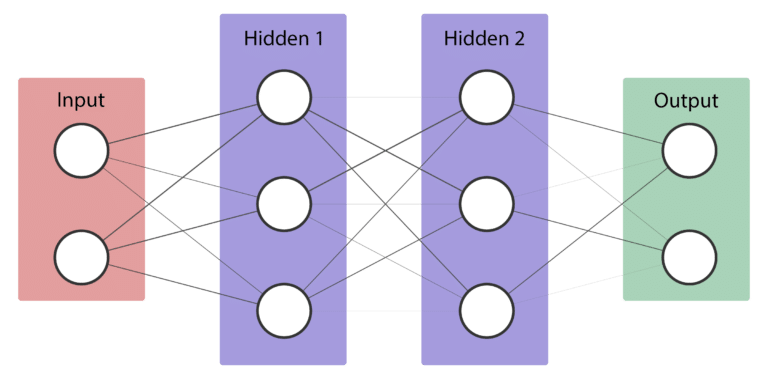# Implementing a flexible neural networkImplementing your own neural network can be hard, especially if you're like me, coming from a computer science background, math equations/syntax makes you dizzy.

Implementing your own neural network can be hard, especially if you're like me, coming from a computer science background, math equations/syntax makes you dizzy and you would understand things better using actual code.

Today I'll show you how easy it is to implement a flexible neural network and train it using the backpropagation algorithm. I'll be implementing this in Python using only NumPy as an external library.

After reading this post, you should understand the following:

• How to feed forward inputs to a neural network.
• Use the Backpropagation algorithm to train a neural network.
• Use the neural network to solve a problem.

In this post, we'll use our neural network to solve a very simple problem: Binary AND.

The code source of the implementation is available here.

### Background knowledge

In order to easily follow and understand this post, you'll need to know the following:

• The basics of Python / OOP.
• An idea of calculus (e.g. dot products, derivatives).
• An idea of neural networks.
• (Optional) How to work with NumPy.

Rest assured though, I'll try to explain everything I do/use here.

## Neural network: what is a neural network?

Neural networks, as their name implies, are computer algorithms modeled after networks of neurons in the human brain. Learn more about neural networks from Algorithmia.

## A Comparative Analysis of Recurrent Neural Networks

Recurrent neural networks, also known as RNNs, are a class of neural networks that allow previous outputs to be used as inputs while having hidden states.

## Recurrent Neural Networks for Multilabel Text Classification Tasks

The purpose of this project is to build and evaluate Recurrent Neural Networks(RNNs) for sentence-level classification tasks. Let's understand about recurrent neural networks for multilabel text classification tasks.

## Neural Network on Beer Dataset

Artificial neural networks (ANNs), usually simply called neural networks (NNs), are computing systems vaguely inspired by the biological neural networks that constitute animal brains.

## Convolutional Neural Network: How is it different from the other networks?

Convolutional Neural Network: How is it different from the other networks? What’s so unique about CNNs and what does convolution really do? This is a math-free introduction to the wonders of CNNs.